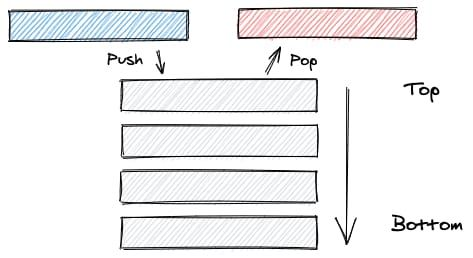# The Stack

Nicolas Toulemont - May 19th 2020
Data Structures

## What is a Stack ?

A Stack is a "last in, first out" (LIFO) data structure where the last inserted element is the first removed. It has two core operations :

• Push : insert an element to the collection
• Pop : remove the most recently inserted element

The Stack also has what is commonly called a "Top" and a "Bottom" :Both the push and pop operations only happen at the "Top" of the Stack (unless the stack length is one, in which case the Top and Bottom are the same).

### Benefits

A stack is very efficient for insertion and deletion of the top item as there is no needed operations with the rest of the stack. Therefore push and pop operations are O(1).

### Downsides

A searching a stack is not efficient as it requires to pop elements of collection until the search target is found. Such operation can then be O(n) is the search target is at the bottom.

### Practical uses in web development

A good example of a stack is the browser navigation history where both back and forward operations return the last page visited.

## Basic methods

We will implement our Stack using Typescript class syntax and an empty object to store our data but an array can also be used.

``````export class Stack<T> {
storage: { [key: number]: T }
length: number
constructor() {
this.storage = {}
this.length = 0
}
}``````
• Push()

The push insert the given value in the object with a key equal to the current length of the stack (therefore, at the very top of it) and increment the stack length.

`````` push(value: T) {
this.storage[this.length] = value;
this.length++;
}``````
• Pop()

The pop get the last index, test its validity, find and store the current last item, delete it from the stack, then decrement the stack length and return the stored last item

`````` pop() {
const lastIndex = this.length - 1;
if (lastIndex < 0) return null;
const lastValue = this.storage[lastIndex];
delete this.storage[lastIndex];
this.length--;
return lastValue;
}``````
• Peek()

The peek get the last item and return correspond item in storage or undefined if none is found

``````peek() {
const lastIndex = this.length - 1;
return this.storage[lastIndex];
}``````

## Helpers methods

Helpers methods such as clear, isEmpty, and print can also be added.

``````  clear() {
if (this.storage === {}) return;
for (const key in this.storage) {
delete this.storage[key];
this.length--;
}
}

isEmpty() {
return this.length === 0;
}

print() {
if (this.storage === {}) {
console.log('Empty stack');
} else {
for (const key in this.storage) {
console.log(`\${key} : \${this.storage[key]}`);
}
}
}``````

• Sort()

This method sort the stack using a recursion during which we recursively traverse the stack and store the top value. This value is then inserted at the top of the stack if is empty or its value is above the current top value.

If the value cannot be pushed at the top of the stack, the current top value (temp) is popped out, the insert function is then recursively called to continue traversing the stack (without its current top value) with the given value to find its position. The current (temps) top value is then pushed back into the stack.

``````  sort(stack: Stack<T>) {
if (!stack.isEmpty()) {
const temp = stack.pop();
this.sort(stack);
this.sortedInsert(stack, temp as T);
}
}

sortedInsert(stack: Stack<T>, item: T) {
if (stack.isEmpty() || item > stack.peek()) {
stack.push(item);
} else {
const temp = stack.pop();
this.sortedInsert(stack, item);
stack.push(temp as T);
}
}``````
• Reverse()

This method uses a recursive insertAtBottom function and follow a similar logic that the sort function, except is doesn't perform a value check in the insertAtBottom function.

``````reverse(stack: Stack<T>) {
if (!stack.isEmpty()) {
const temp = stack.pop();
this.reverse(stack);
this.insertAtBottom(stack, temp as T);
}
}

insertAtBottom(stack: Stack<T>, item: T) {
if (stack.isEmpty()) {
stack.push(item);
} else {
const temp = stack.pop();
this.insertAtBottom(stack, item);
stack.push(temp as T);
}
}``````